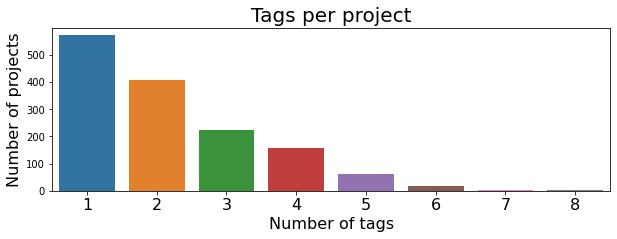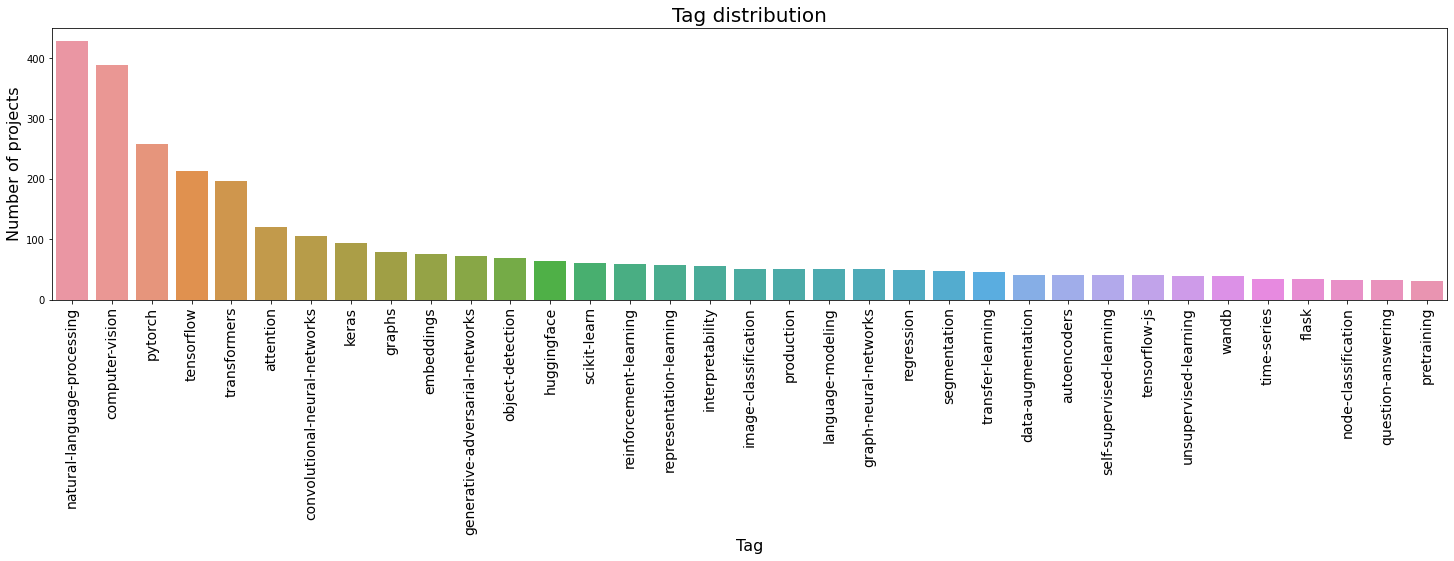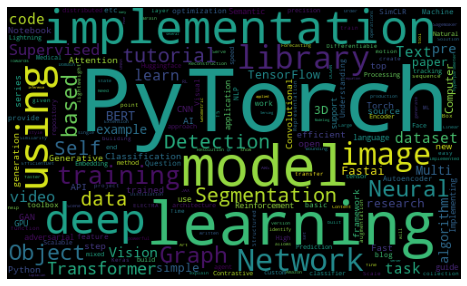# Exploratory data analysis

Exploring our dataset for insights with intention.

## Intuition

Exploratory data analysis (EDA) is a vital (and fun) step in the data science process but it's often misconstrued. Here's how to think about EDA:

• not just to visualize a prescribed set of plots (correlation matrix, etc.).
• goal is to convince yourself that the data you currently have is sufficient for the task.
• use EDA to answer questions and ask yourself why those questions are important.
• not a one time process; as your data grows, you want to revisit EDA to catch distribution shifts, anomalies, etc.

## Application

 1 2 3 4 5 import matplotlib.pyplot as plt import seaborn as sns import warnings from wordcloud import WordCloud, STOPWORDS warnings.filterwarnings("ignore") 

### Tags per project

Q1. How many (post filtered) tags do the projects have? We care about this because we want to make sure we don't overwhelm the user with too many tags (UX constraint).

  1 2 3 4 5 6 7 8 9 10 # Number of tags per project num_tags_per_project = [len(tags) for tags in df.tags] num_tags, num_projects = zip(*Counter(num_tags_per_project).items()) plt.figure(figsize=(10, 3)) ax = sns.barplot(list(num_tags), list(num_projects)) plt.title("Tags per project", fontsize=20) plt.xlabel("Number of tags", fontsize=16) ax.set_xticklabels(range(1, len(num_tags)+1), rotation=0, fontsize=16) plt.ylabel("Number of projects", fontsize=16) plt.show()### Tag distribution

Q2. What are the most popular tags? We care about this because it's important to know about the distribution of tags and what tags just made the cut (for performance).

  1 2 3 4 5 6 7 8 9 10 # Distribution of tags all_tags = list(itertools.chain.from_iterable(df.tags.values)) tags, tag_counts = zip(*Counter(all_tags).most_common()) plt.figure(figsize=(25, 5)) ax = sns.barplot(list(tags), list(tag_counts)) plt.title("Tag distribution", fontsize=20) plt.xlabel("Tag", fontsize=16) ax.set_xticklabels(tags, rotation=90, fontsize=14) plt.ylabel("Number of projects", fontsize=16) plt.show()### Wordcloud

Q3. Is there enough signal in the title and description that's unique to each tag? This is important because we want to verify our initial hypothesis that the project's title and description are highly influential features.

  1 2 3 4 5 6 7 8 9 10 11 @widgets.interact(tag=list(tags)) def display_word_cloud(tag='pytorch'): # Plot word clouds top top tags plt.figure(figsize=(15, 5)) subset = df[df.tags.apply(lambda tags: tag in tags)] text = subset.text.values cloud = WordCloud( stopwords=STOPWORDS, background_color='black', collocations=False, width=500, height=300).generate(" ".join(text)) plt.axis('off') plt.imshow(cloud)Note

All of the work we've done so far are inside IPython notebooks but in a later lesson, we'll transfer all of this into an interactive dashboard using a tool called Streamlit.